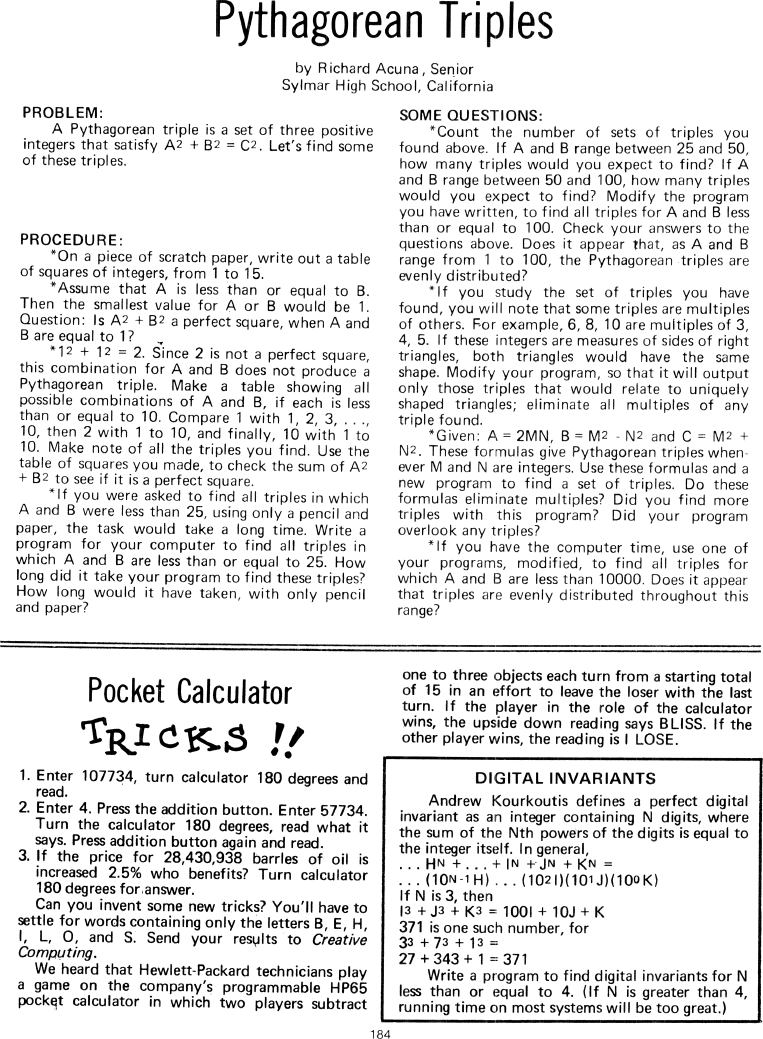The Best of Creative Computing Volume 1 (published 1976)

 `Page 184 << PREVIOUS >> NEXT Jump to page: Go to contents Go to thumbnails`

Pythagorean Triples (math puzzles)
Pocket Calculator Tricks (spelling words with calculators)```Pythagorean Triples
by Richard Acuna, Senior
Sylmar High School, California

PROBLEM:

A Pythagorean triple is a set of three positive integers that satisfy A2 + B2 =
C2. Let's find some of these triples.

PROCEDURE:

*On a piece of scratch paper, write out a table of squares of integers, from 1
to 15.

*Assume that A is less than or equal to B. Then the smallest value for A or B
would be 1. Question: Is A2 + B2 a perfect square, when A and B are equal to 1?
*12 + 12 = 2. Since 2 is not a perfect square, this combination for A and B does
not produce a Pythagorean triple. Make a table showing all possible combinations
of A and B, if each is less than or equal to 10. Compare 1 with 1, 2, 3 ,...
,10, then 2 with 1 to 10, and finally, 10 with 1 to 10. Make note of all the
triples you find. Use the table of squares you made, to check the sum of A2 + B2
to see if it is a perfect square.

*If you were asked to find all triples in which A and B were less than 25, using
only a pencil and paper, the task would take a long time. Write a program for
your computer to find all triples in which A and B are less than or equal to 25.
How long did it take your program to find these triples? How long would it have
taken, with only pencil and paper?

SOME QUESTIONS:

*Count the number of sets of triples you found above. If A and B range between
25 and 50, how many triples would you expect to find? If A and B range between
50 and 100, how many triples would you expect to find? Modify the program you
have written, to find all triples for A and B less than or equal to 100. Check
your answers to the questions above. Does it appear that, as A and B range from
1 to 100, the Pythagorean triples are evenly distributed?

*If you study the set of triples you have found, you will note that some triples
are multiples of others. For example, 6, 8, 10 are multiples of 3, 4, 5. If
these integers are measures of sides of right triangles, both triangles would
have the same shape. Modify your program, so that it will output only those
triples that would relate to uniquely shaped triangles; eliminate all multiples
of any triple found.

*Given: A= 2MN, B = M2 - N2 and C = M2 + N2. These formulas give Pythagorean
triples whenever M and N are integers. Use these formulas and a new program to
find a set of triples. Do these formulas eliminate multiples? Did you find more
triples with this program? Did your program overlook any triples?

*If you have the computer time, use one of your programs, modified, to find all
triples for which A and B are less than 10000. Does it appear that triples are
evenly distributed throughout this range?

**************************************************************************************************************
Pocket Calculator
TRICKS !!

1. Enter 107734, turn calculator 180 degrees and read.

2. Enter 4. Press the addition button. Enter 57734. Turn the calculator 180

3. If the price for 28,430,938 barrles of oil is increased 2.5% who benefits?
Turn calculator 180 degrees for answer.

Can you invent some new tricks? You'll have to settle for words containing only
the letters B, E, H, I, L, O, and S. Send your results to Creative Computing.

We heard that Hewlett-Packard technicians play a game on the company's
programmable HP65 pocket calculator in which two players subtract one to three
objects each turn from a starting total of 15 in an effort to leave the loser
with the last turn. If the player in the role of the calculator wins, the upside
down reading says BLISS. If the other player wins, the reading is I LOSE.

DIGITAL INVARIANTS

Andrew Kourkoutis defines a perfect digital invariant as an integer containing N
digits, where the sum of the Nth powers of the digits is equal to the integer
itself. In general,
...HN +...+lN +JN +KN = ...(10N-1H)...(102I)(101J)(100K)
If N is 3, then I3+J3+K3 =100I+10J+K
371 is one such number, for 33 + 73 + 13 =27+343+1=371

Write a program to find digital invariants for N less than or equal to 4. (If N
is greater than 4, running time on most systems will be too great.)Page 184        << PREVIOUS        >> NEXT        Jump to page:                Go to contents        Go to thumbnails

<!--